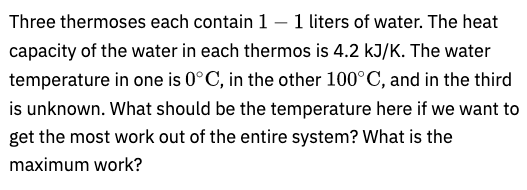# Thermodynamics problem based on three thermoses

Please provide a solution for this problem. I seem to be making some error etc.@Alisher @Ersultan @Miras @Damir and anyone else who wants to help.

This is the first time I’ve seen a problem, where you most likely need to use the matching method. Since the thermos is insulated, the work will change depending on the change of internal energy in the thermos. So, we need to find the case, where the internal energy changes the least, because according to the formula:

Q=\Delta U+A=0 \Rightarrow \\ \Rightarrow\Delta U=-A

for the maximum work we need the minimum change of internal energy.

2 лайка

ok so what answer are you getting?

Since the total entropy change is zero, \displaystyle dS =C\left( \frac{dT_1}{T_1}+ \frac{dT_2}{T_2} + \frac{dT_3}{T_3} \right) =0 . Therefore, we will have T_1T_2T_3 = T_{01}T_{02}T_{03} for the process.

The engine will run till all the temperatures will be equal, so T_f = (T_{01}T_{02}T_{03})^{\frac{1}{3}}.

As Miras mentioned,

So the total work will be A =C(T_{01}+T_{02}+T_{03} - 3 (T_{01}T_{02}T_{03})^{\frac{1}{3}}). For maximum work we will get T_{03} = \sqrt{T_{01}T_{02}}=319 K, and A_{max} =C (\sqrt{T_{01}}- \sqrt{T_{02}})^2 = 32.7 kJ

5 лайков

Thank you so much!

Where would you rate the difficulty of this question? This was from Rudolf Ortvay 1994 (not available publicly).

I would say that it is a somewhat standard problem on entropy

3 лайка

oh ok… thank you!

Did you read Blundell on this topic?

1 лайк

No not yet. Well, I have read one page but not properly

Just read thoroughly about the second thermodynamics law – it is super important.

1 лайк

Ok. I’ll read it next year… haha